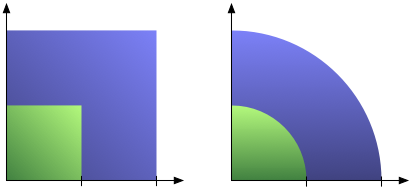# Linear vs. Quadratic Change ​

One of the most common mistakes in chart design is to scale an area by two sides at the same time, producing a quadratic effect for a linear change. That overstates the larger numbers and produces a badly skewed chart. A little care and some basic high-school math can help avoid the problem.

The following detail from a information graphic produced by Princeton's International Networks Archive illustrates the problem (the numbers are presumably from 2002):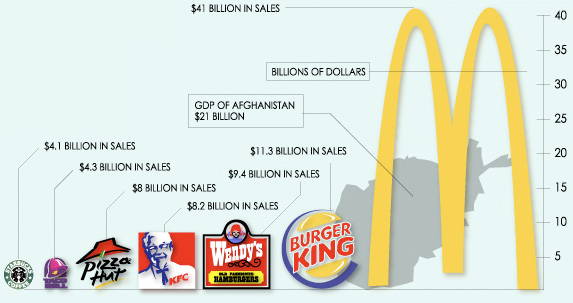Comparing Starbucks (\$4.1bn) and KFC (\$8.2bn), the problem becomes clear: the difference is a factor of two, but the KFC logo has four times the area of the Starbucks logo (even more because one is square and the other round). This can be seen in a number of the graphics on that website, though they also have some where they scale correctly.

The reason for the problem here is the use of logos (or of images, more generally) to make charts look better. Scaling a logo in only one dimension (which would be done in a bar chart) does not work because the image would look stretched and ugly. So instead, the image is scaled in two dimensions, leading to a perceived difference that is the square of the actual difference.

In more general terms, a linear change (I will use a factor of two to illustrate this)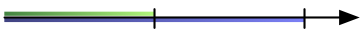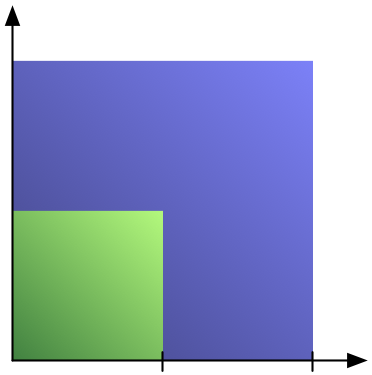Or, to employ a bit of high-school math: The area of a square A = a2, with a being the length of side of the square. If we double the side length, we get A' = (2a)2 = 4a2: four times the area. This is the case for many other shapes as well, including circles and circle segments.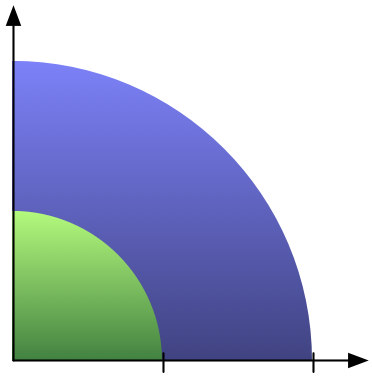That last part was actually the subject of a discussion I had a while ago with a rather senior visualization person. He did not believe that changing a circle segment's radius would lead to a quadratic increase in its area. It's easy to show, though: a circle's area is r2π (r being the circle's radius), the area of a circle segment that covers an angle θ is r2π·sin(θ). It is no more difficult to show that doubling the radius will quadruple the area than with the square above.

Florence Nightingale already knew this in 1858 when she developed her coxcomb chart (a predecessor to the pie chart): she represented the numbers of soldiers using the area, not the radius, of the circle segments.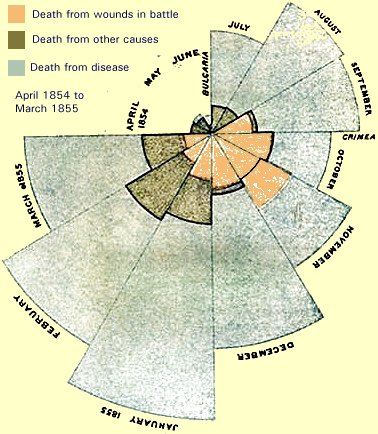A similar effect can be seen in the petal chart or star glyph, which connects points on a number of axes that radiate from a common point. Whether they are filled in or not, the impression is that of an enclosed area, and that changes in a quadratic way similar to the circle segments above.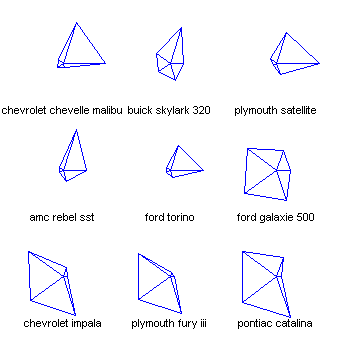The solution? Either use a better visualization (star glyphs in particular are very difficult to read) or scale your circles, squares, and other lengths by the square root of the value you want to represent. That makes the area scale linearly with the value that is to be represented.

Posted by Robert Kosara on September 19, 2008.